# Condensed Matter Collaborations 1999 Network

Weighted network of collaborations between scientists posting preprints

Weighted network of collaborations between scientists posting preprints on the Condensed Matter E-Print Archive between Jan 1, 1995 and December 31, 1999 with edge weight indicating the strength of the collaboration between pairs of individuals.

## Examples

### Basic Examples

Retrieve the graph:

 In:=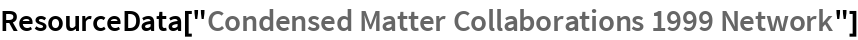Out=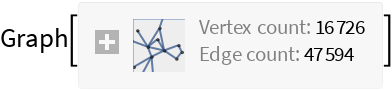Summary properties:

 In:=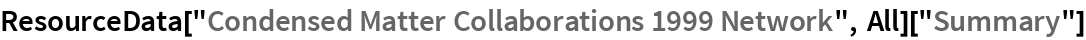Out=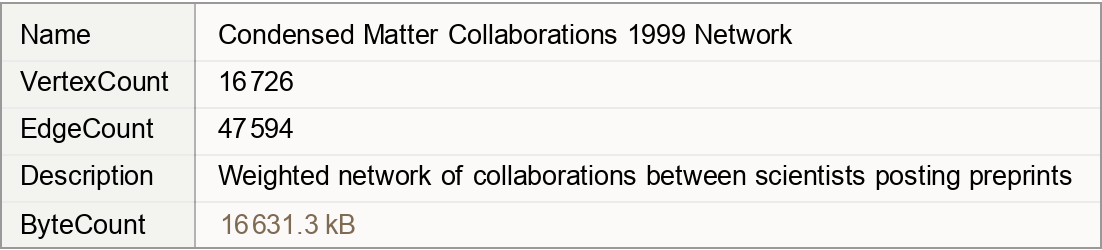### Basic Applications

Pick the author with the most collaborations:

 In:=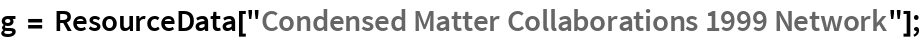In:=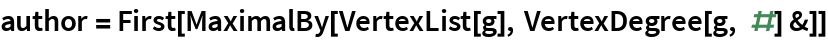Out=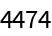Visualize the network around the author with the most collaborations:

 In:=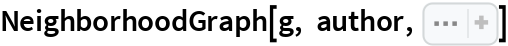Out=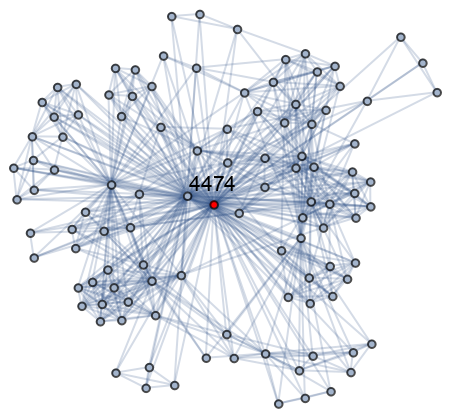Wolfram Research, "Condensed Matter Collaborations 1999 Network" from the Wolfram Data Repository (2019)